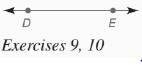Chapter 7.CR, Problem 10CRElementary Geometry For College St...

7th Edition
Alexander + 2 others
ISBN: 9781337614085

Solutions

Chapter
SectionElementary Geometry For College St...

7th Edition
Alexander + 2 others
ISBN: 9781337614085
Textbook Problem

In Review Exercises 7 to 13, sketch and describe the locus in a plane.Find the locus of points that are 1 2 in. from D E ↔ .To determine

To find: The locus of points that are 12 inch from DE.

Explanation

Given:

The line with points D and E is shown below

Definition used:

A locus is the set of all points and only those points that satisfy a given condition (or set of conditions).

Calculation:

Consider the given figure.

The points that are 12 inch from DE are the two parallel lines in either side of DE.

That is, each line that are 12 inch from DE and the opposite of it

Still sussing out bartleby?

Check out a sample textbook solution.

See a sample solution

The Solution to Your Study Problems

Bartleby provides explanations to thousands of textbook problems written by our experts, many with advanced degrees!

Get Started

Evaluate the expression sin Exercises 116. 3(2)0

Finite Mathematics and Applied Calculus (MindTap Course List)

Find the limit or show that it does not exist. limx(2x21)2(x1)2(x2+x)

Single Variable Calculus: Early Transcendentals, Volume I

Find dy/dx by implicit differentiation. 8. x3 xy2 + y3 = 1

Single Variable Calculus: Early Transcendentals

True or False: converges mean exists.

Study Guide for Stewart's Multivariable Calculus, 8th

ex(ex)2 = a) ex3 b) e3x c) 3ex d) e4x

Study Guide for Stewart's Single Variable Calculus: Early Transcendentals, 8th

In Exercises 1-8, construct a truth table for each compound proposition. p~q

Finite Mathematics for the Managerial, Life, and Social Sciences